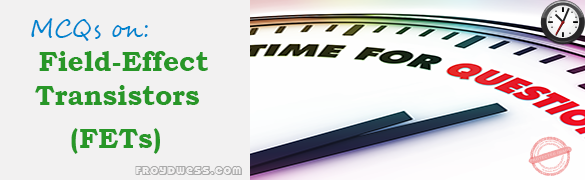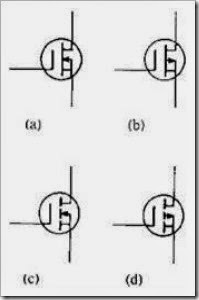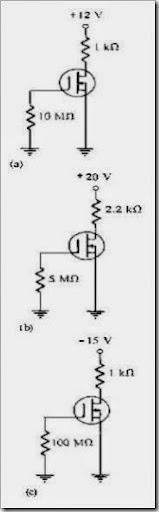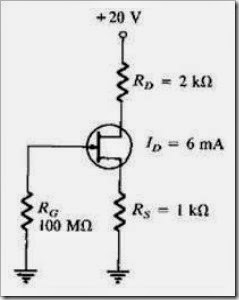# MCQs in Field-Effect Transistors (FETs)

(Last Updated On: December 8, 2017)This is the Multiple Choice Questions in Field-Effect Transistors (FETs) from the book Electronic Devices – Electron Flow Version and Conventional Current Version 8th Edition by Thomas L. Floyd. If you are looking for a reviewer in Electronics Engineering this will definitely help. I can assure you that this will be a great help in reviewing the book in preparation for your Board Exam. Make sure to familiarize each and every questions to increase the chance of passing the ECE Board Exam.

### Online Questions and Answers Topic Outline

• MCQs in Field-Effect Transistors (FETs) | MCQs in JFET | MCQs in JFET Characteristics and Parameters | MCQs in JFET Biasing | MCQs in The Ohmic Region | MCQs in MOSFET | MCQs in MOSFET Characteristics and Parameters | MCQs in MOSFET Biasing | MCQs in IGBT

### Practice Exam Test Questions

Choose the letter of the best answer in each questions.

1. On the drain characteristic curve of a JFET for VGS = 0, the pinch-off voltage is
• A) below the ohmic area.
• B) between the ohmic area and the constant current area.
• C) between the constant current area and the breakdown region.
• D) above the breakdown region.
2. For a JFET, the value of VDS at which ID becomes essentially constant is the
• A) pinch-off voltage.
• B) cutoff voltage.
• C) breakdown voltage.
• D) ohmic voltage.

3. The value of VGS that makes ID approximately zero is the

• A) pinch-off voltage.
• B) cutoff voltage.
• C) breakdown voltage.
• D) ohmic voltage.
4. For a JFET, the change in drain current for a given change in gate-to-source voltage, with the drain-to-source voltage constant, is
• A) breakdown.
• B) reverse transconductance.
• C) forward transconductance.
• D) self-biasing.

5. High input resistance for a JFET is due to

• A) a metal oxide layer.
• B) a large input resistor to the device.
• C) an intrinsic layer.
• D) the gate-source junction being reverse-biased.

6. A dual-gated MOSFET is

• A) a depletion MOSFET.
• B) an enhancement MOSFET.
• C) a VMOSFET.
• D) either a depletion or an enhancement MOSFET.

7. Which of the following devices has the highest input resistance?

• A) diode
• B) JFET
• C) MOSFET
• D) bipolar junction transistor
8. A self-biased n-channel JFET has a VD = 6 V. VGS = –3 V. Find the value of VDS.
• A) –3 V
• B) –6 V
• C) 3 V
• D) 6 V

9. Refer to Figure 8-1. Identify the p-channel E-MOSFET.Figure 8-1

• A) a
• B) b
• C) c
• D) d

10. Refer to Figure 8-1. Identify the n-channel D-MOSFET.

• A) a
• B) b
• C) c
• D) d

11. Refer to Figure 8-1. Identify the n-channel E-MOSFET.

• A) a
• B) b
• C) c
• D) d

12. Refer to Figure 8-1. Identify the p-channel D-MOSFET.

• A) a
• B) b
• C) c
• D) d

13. Refer to Figure 8-2(a). ID = 6 mA. Calculate the value of VDS.Figure 8-2

• A) –6 V
• B) 6 V
• C) 12 V
• D) –3 V

14. Refer to Figure 8-2(b). ID = 6 mA. Calculate the value of VDS.

• A) 13.2 V
• B) 10 V
• C) 6.8 V
• D) 0 V

15. Refer to Figure 8-2(c). ID = 6 mA. Calculate the value of VDS.

• A) –9 V
• B) 9 V
• C) 6 V
• D) –3 V
16. A JFET data sheet specifies VGS(off) = –6 V and IDSS = 8 mA. Find the value of ID when VGS = –3 V.
• A) 2 mA
• B) 4 mA
• C) 8 mA
• D) none of the above
17. A JFET data sheet specifies VGS(off) = –10 V and IDSS = 8 mA. Find the value of ID when VGS = –3 V.
• A) 2 mA
• B) 1.4 mA
• C) 4.8 mA
• D) 3.92 mA

18. Refer to Figure 8-3. Determine the value of VS.Figure 8-3

• A) 20 V
• B) 8 V
• C) 6 V
• D) 2 V

19. Refer to Figure 8-3. Calculate the value of VD.

• A) 20 V
• B) 8 V
• C) 6 V
• D) 2 V

20. Refer to Figure 8-3. What is the value of IG?

• A) 6 mA
• B) 4 mA
• C) 2 mA
• D) 0 mA

21. Refer to Figure 8-3. Determine the value of VGS.

• A) –20 V
• B) –8 V
• C) –6 V
• D) –2 V

22. Refer to Figure 8-3. Calculate the value of VDS.

• A) 0 V
• B) 2 V
• C) 4 V
• D) –2 V
23. The JFET is always operated with the gate-source pn junction _____ -biased.
• A) forward
• B) reverse
24. What three areas are the drain characteristics of a JFET (VGS = 0) divided into?
• A) ohmic, constant-current, breakdown
• B) pinch-off, constant-current, avalanche
• C) ohmic, constant-voltage, breakdown
25. What type(s) of gate-to-source voltage(s) can a depletion MOSFET (D-MOSFET) operate with?
• A) zero
• B) positive
• C) negative
• D) any of the above

26. The _____ has a physical channel between the drain and source.

• A) D-MOSFET
• B) E-MOSFET
• C) V-MOSFET
27. All MOSFETs are subject to damage from electrostatic discharge (ESD).
• A) true
• B) false
28. Midpoint bias for a D-MOSFET is ID = _____, obtained by setting VGS = 0.
• A) IDSS / 2
• B) IDSS / 3.4
• C) IDSS

29. In a self-biased JFET circuit, if VD = VDD then ID = _____.

• A) 0
• B) cannot be determined from information above
30. If VD is less than expected (normal) for a self-biased JFET circuit, then it could be caused by a(n)
• A) open RG.
• B) open gate lead.
• C) FET internally open at gate.
• D) all of the above

31. The resistance of a JFET biased in the ohmic region is controlled by

• A) VD.
• B) VGS.
• C) VS.
• D) VDS.

### Complete List of Chapter MCQs in Floyd’s Electronic Devices

credit: © 2014 www.PinoyBIX.org
Rate this: